# Search by Topic

#### Resources tagged with Odd and even numbers similar to Birthday Cakes:

Filter by: Content type:
Age range:
Challenge level:

### There are 64 results

Broad Topics > Numbers and the Number System > Odd and even numbers### Pairs of Legs

##### Age 5 to 7 Challenge Level:

How many legs do each of these creatures have? How many pairs is that?### How Odd

##### Age 5 to 7 Challenge Level:

This problem challenges you to find out how many odd numbers there are between pairs of numbers. Can you find a pair of numbers that has four odds between them?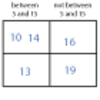### More Carroll Diagrams

##### Age 7 to 11 Challenge Level:

How have the numbers been placed in this Carroll diagram? Which labels would you put on each row and column?### Carroll Diagrams

##### Age 5 to 11 Challenge Level:

Use the interactivities to fill in these Carroll diagrams. How do you know where to place the numbers?### Cube Bricks and Daisy Chains

##### Age 5 to 7 Challenge Level:

Daisy and Akram were making number patterns. Daisy was using beads that looked like flowers and Akram was using cube bricks. First they were counting in twos.### What Number?

##### Age 5 to 7 Short Challenge Level:

I am less than 25. My ones digit is twice my tens digit. My digits add up to an even number.### Light the Lights

##### Age 5 to 7 Challenge Level:

Investigate which numbers make these lights come on. What is the smallest number you can find that lights up all the lights?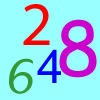### Largest Even

##### Age 5 to 7 Challenge Level:

How would you create the largest possible two-digit even number from the digit I've given you and one of your choice?### The Set of Numbers

##### Age 5 to 7 Challenge Level:

Can you place the numbers from 1 to 10 in the grid?### Arrangements

##### Age 7 to 11 Challenge Level:

Is it possible to place 2 counters on the 3 by 3 grid so that there is an even number of counters in every row and every column? How about if you have 3 counters or 4 counters or....?### The Thousands Game

##### Age 7 to 11 Challenge Level:

Each child in Class 3 took four numbers out of the bag. Who had made the highest even number?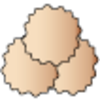### Lots of Biscuits!

##### Age 5 to 7 Challenge Level:

Help share out the biscuits the children have made.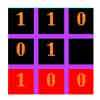### Odd Tic Tac

##### Age 5 to 7 Challenge Level:

An odd version of tic tac toe### I Like ...

##### Age 5 to 7 Challenge Level:

Mr Gilderdale is playing a game with his class. What rule might he have chosen? How would you test your idea?### Grouping Goodies

##### Age 5 to 7 Challenge Level:

Pat counts her sweets in different groups and both times she has some left over. How many sweets could she have had?### One of Thirty-six

##### Age 5 to 7 Challenge Level:

Can you find the chosen number from the grid using the clues?### Seven Flipped

##### Age 7 to 11 Challenge Level:

Investigate the smallest number of moves it takes to turn these mats upside-down if you can only turn exactly three at a time.### Number Detective

##### Age 5 to 11 Challenge Level:

Follow the clues to find the mystery number.### Odds and Threes

##### Age 7 to 11 Challenge Level:

A game for 2 people using a pack of cards Turn over 2 cards and try to make an odd number or a multiple of 3.### Part the Piles

##### Age 7 to 11 Challenge Level:

Try to stop your opponent from being able to split the piles of counters into unequal numbers. Can you find a strategy?### Two Numbers Under the Microscope

##### Age 5 to 7 Challenge Level:

This investigates one particular property of number by looking closely at an example of adding two odd numbers together.### Odd Times Even

##### Age 5 to 7 Challenge Level:

This problem looks at how one example of your choice can show something about the general structure of multiplication.### Venn Diagrams

##### Age 5 to 11 Challenge Level:

Use the interactivities to complete these Venn diagrams.### Curious Number

##### Age 7 to 11 Challenge Level:

Can you order the digits from 1-3 to make a number which is divisible by 3 so when the last digit is removed it becomes a 2-figure number divisible by 2, and so on?### Number Round Up

##### Age 5 to 7 Challenge Level:

Arrange the numbers 1 to 6 in each set of circles below. The sum of each side of the triangle should equal the number in its centre.### Becky's Number Plumber

##### Age 7 to 11 Challenge Level:

Becky created a number plumber which multiplies by 5 and subtracts 4. What do you notice about the numbers that it produces? Can you explain your findings?### Play to 37

##### Age 7 to 11 Challenge Level:

In this game for two players, the idea is to take it in turns to choose 1, 3, 5 or 7. The winner is the first to make the total 37.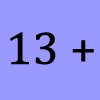### What Could it Be?

##### Age 5 to 7 Challenge Level:

In this calculation, the box represents a missing digit. What could the digit be? What would the solution be in each case?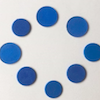### Eightness of Eight

##### Age 5 to 7 Challenge Level:

What do you see as you watch this video? Can you create a similar video for the number 12?### Always, Sometimes or Never?

##### Age 5 to 11 Challenge Level:

Are these statements relating to odd and even numbers always true, sometimes true or never true?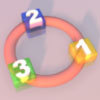### More Numbers in the Ring

##### Age 5 to 7 Challenge Level:

If there are 3 squares in the ring, can you place three different numbers in them so that their differences are odd? Try with different numbers of squares around the ring. What do you notice?### What Do You Need?

##### Age 7 to 11 Challenge Level:

Four of these clues are needed to find the chosen number on this grid and four are true but do nothing to help in finding the number. Can you sort out the clues and find the number?### Ring a Ring of Numbers

##### Age 5 to 7 Challenge Level:

Choose four of the numbers from 1 to 9 to put in the squares so that the differences between joined squares are odd.### Next-door Numbers

##### Age 5 to 7 Challenge Level:

Florence, Ethan and Alma have each added together two 'next-door' numbers. What is the same about their answers?### Numbers as Shapes

##### Age 5 to 7 Challenge Level:

Use cubes to continue making the numbers from 7 to 20. Are they sticks, rectangles or squares?### Multiplication Series: Number Arrays

##### Age 5 to 11

This article for teachers describes how number arrays can be a useful reprentation for many number concepts.### Domino Sorting

##### Age 5 to 7 Challenge Level:

Try grouping the dominoes in the ways described. Are there any left over each time? Can you explain why?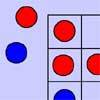### Red Even

##### Age 7 to 11 Challenge Level:

You have 4 red and 5 blue counters. How many ways can they be placed on a 3 by 3 grid so that all the rows columns and diagonals have an even number of red counters?### Sets of Four Numbers

##### Age 7 to 11 Challenge Level:

There are ten children in Becky's group. Can you find a set of numbers for each of them? Are there any other sets?### Share Bears

##### Age 5 to 7 Challenge Level:

Yasmin and Zach have some bears to share. Which numbers of bears can they share so that there are none left over?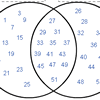### Various Venns

##### Age 7 to 11 Challenge Level:

Use the interactivities to complete these Venn diagrams.### Down to Nothing

##### Age 7 to 11 Challenge Level:

A game for 2 or more people. Starting with 100, subratct a number from 1 to 9 from the total. You score for making an odd number, a number ending in 0 or a multiple of 6.### A Mixed-up Clock

##### Age 7 to 11 Challenge Level:

There is a clock-face where the numbers have become all mixed up. Can you find out where all the numbers have got to from these ten statements?### Sorting Numbers

##### Age 5 to 7 Challenge Level:

Can you sort numbers into sets? Can you give each set a name?### Make 37

##### Age 7 to 14 Challenge Level:

Four bags contain a large number of 1s, 3s, 5s and 7s. Pick any ten numbers from the bags above so that their total is 37.### Square Subtraction

##### Age 7 to 11 Challenge Level:

Look at what happens when you take a number, square it and subtract your answer. What kind of number do you get? Can you prove it?### Sets of Numbers

##### Age 7 to 11 Challenge Level:

How many different sets of numbers with at least four members can you find in the numbers in this box?### Magic Crosses

##### Age 7 to 14 Challenge Level:

Can you find examples of magic crosses? Can you find all the possibilities?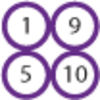### Pairs of Numbers

##### Age 5 to 7 Challenge Level:

If you have ten counters numbered 1 to 10, how many can you put into pairs that add to 10? Which ones do you have to leave out? Why?### Odd Squares

##### Age 7 to 11 Challenge Level:

Think of a number, square it and subtract your starting number. Is the number you’re left with odd or even? How do the images help to explain this?